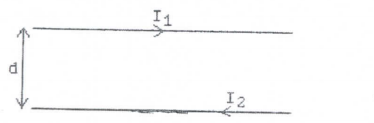# Problem: In the figure below, the two long straight wires are separated by a distance of d = 0.40 m. The currents are I1 = 5.0 A to the right in the upper wire and I 2 = 6.0 A to the left in the lower wire. What is the magnitude and direction of the force on wire 2 due to wire 1 if the wire 1 is 10 cm long?

🤓 Based on our data, we think this question is relevant for Professor Hobbs' class at SUNY.

###### Problem Details

In the figure below, the two long straight wires are separated by a distance of d = 0.40 m. The currents are I1 = 5.0 A to the right in the upper wire and I 2 = 6.0 A to the left in the lower wire. What is the magnitude and direction of the force on wire 2 due to wire 1 if the wire 1 is 10 cm long?